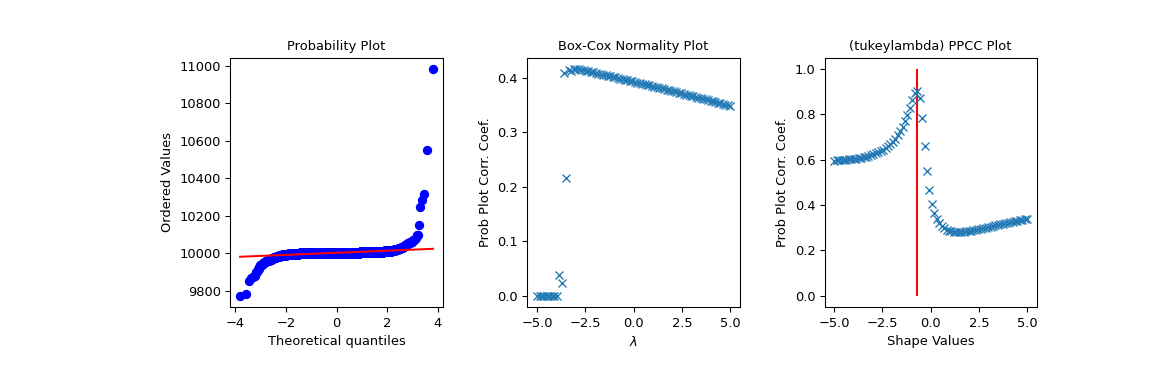# scipy.stats.ppcc_plot¶

scipy.stats.ppcc_plot(x, a, b, dist='tukeylambda', plot=None, N=80)[source]

Calculate and optionally plot probability plot correlation coefficient.

The probability plot correlation coefficient (PPCC) plot can be used to determine the optimal shape parameter for a one-parameter family of distributions. It cannot be used for distributions without shape parameters (like the normal distribution) or with multiple shape parameters.

By default a Tukey-Lambda distribution (stats.tukeylambda) is used. A Tukey-Lambda PPCC plot interpolates from long-tailed to short-tailed distributions via an approximately normal one, and is therefore particularly useful in practice.

Parameters
xarray_like

Input array.

a, bscalar

Lower and upper bounds of the shape parameter to use.

diststr or stats.distributions instance, optional

Distribution or distribution function name. Objects that look enough like a stats.distributions instance (i.e. they have a ppf method) are also accepted. The default is 'tukeylambda'.

plotobject, optional

If given, plots PPCC against the shape parameter. plot is an object that has to have methods “plot” and “text”. The matplotlib.pyplot module or a Matplotlib Axes object can be used, or a custom object with the same methods. Default is None, which means that no plot is created.

Nint, optional

Number of points on the horizontal axis (equally distributed from a to b).

Returns
svalsndarray

The shape values for which ppcc was calculated.

ppccndarray

The calculated probability plot correlation coefficient values.

References

J.J. Filliben, “The Probability Plot Correlation Coefficient Test for Normality”, Technometrics, Vol. 17, pp. 111-117, 1975.

Examples

First we generate some random data from a Tukey-Lambda distribution, with shape parameter -0.7:

>>> from scipy import stats
>>> import matplotlib.pyplot as plt
>>> np.random.seed(1234567)
>>> x = stats.tukeylambda.rvs(-0.7, loc=2, scale=0.5, size=10000) + 1e4


Now we explore this data with a PPCC plot as well as the related probability plot and Box-Cox normplot. A red line is drawn where we expect the PPCC value to be maximal (at the shape parameter -0.7 used above):

>>> fig = plt.figure(figsize=(12, 4))
>>> res = stats.probplot(x, plot=ax1)
>>> res = stats.boxcox_normplot(x, -5, 5, plot=ax2)
>>> res = stats.ppcc_plot(x, -5, 5, plot=ax3)
>>> ax3.vlines(-0.7, 0, 1, colors='r', label='Expected shape value')
>>> plt.show()#### Previous topic

scipy.stats.ppcc_max

#### Next topic

scipy.stats.probplot